### iPhone Screenshots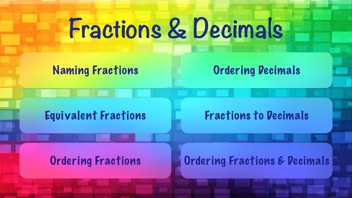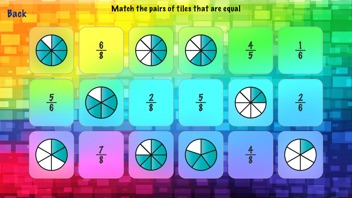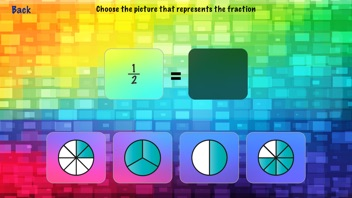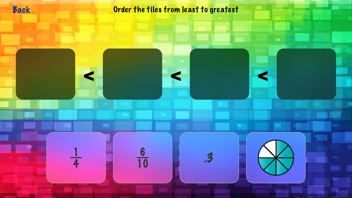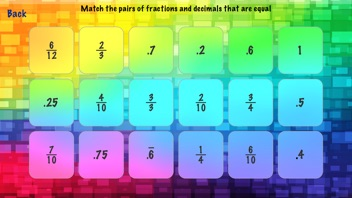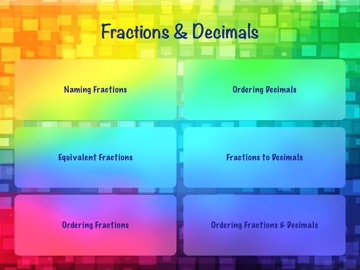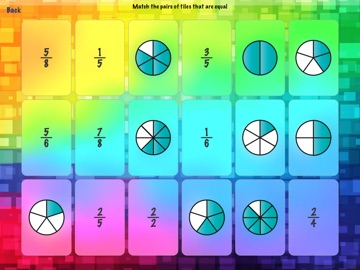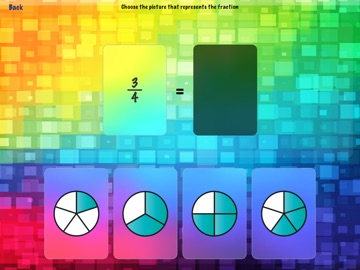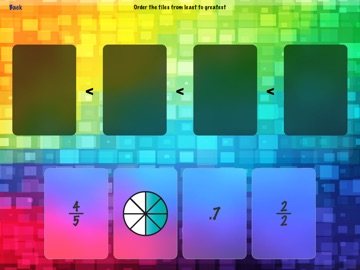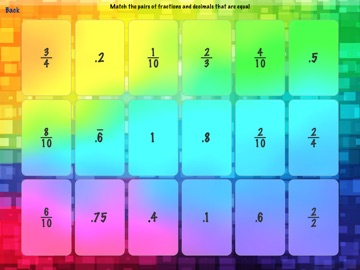### Description

Fractions & Decimals has fun and simple activities to practice a range of fraction and decimal concepts such as: naming fractions, equivalent fractions, ordering fractions and decimals, and equivalent fractions and decimals.
The app has the following activities:
Naming Fractions:
Multiple Choice Mode: Choose the picture that represents the fraction
Matching Mode: Match fractions to the pictures of the fractions
Equivalent Fractions:
Multiple Choice Mode: Choose the fraction equivalent to the given fraction
Matching Mode: Match the pairs of equivalent fractions
Ordering Fractions:
Order fractions from least to greatest (2 levels)
Ordering Decimals:
Order decimals from least to greatest
Fractions to Decimals:
Multiple Choice Mode: Choose the decimal that is equal to the given fraction (2 levels)
Matching Mode: Match the pairs of fractions and decimals that are equal to each other. (2 levels)
Ordering Fractions and Decimals:
Order the fractions, decimals and their picture representations in order from least to greatest (2 levels)

TicTapTech, LLC

1.0

5.8 MB

No

Yes

Rated: 4+

iOS 8.1 or later

English

No rating

No rating

No Entry